# SampleRNN overview

SampleRNN uses recurrent neural networks to find patterns in audio waveforms: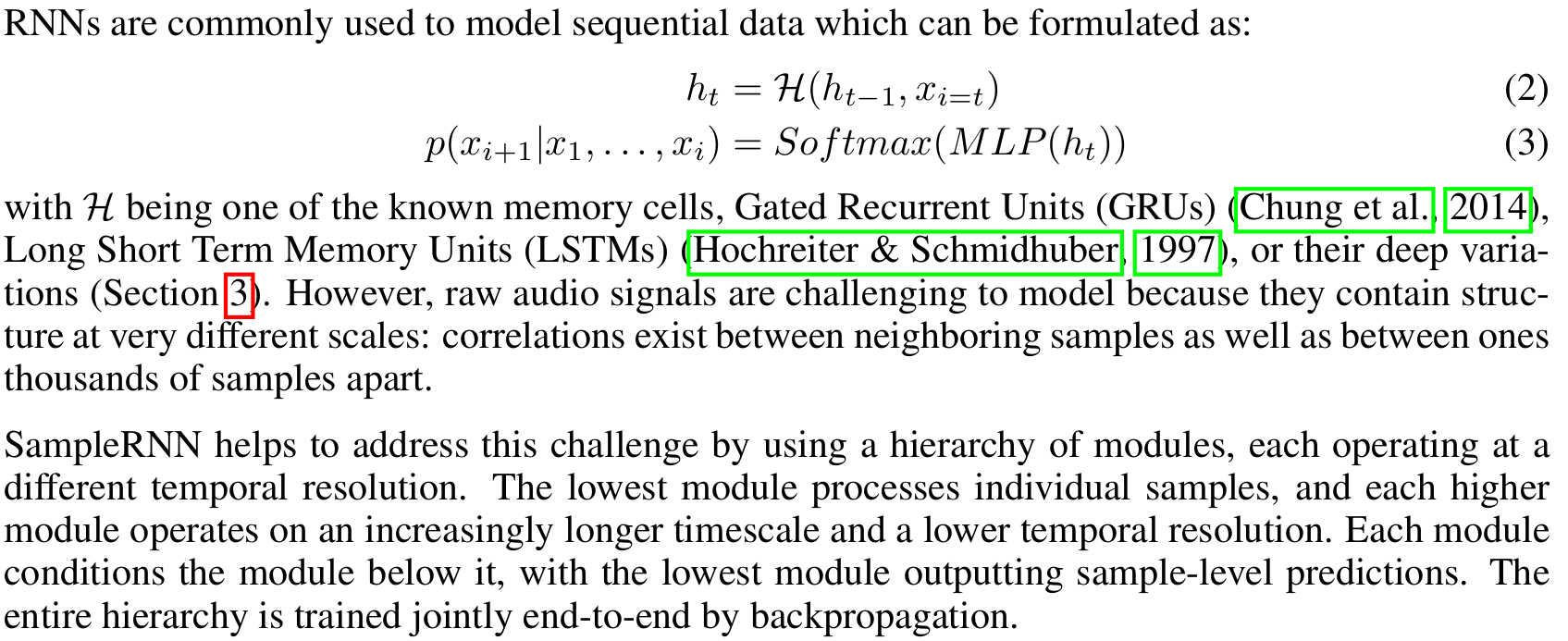Note the important link to the causality of WaveNet's causal convolutions is that the memory cell uses past memory to compute present samples, or `h[t] = H(h[t-1])`.

SampleRNN is an autoregressive model like WaveNet, meaning that it computes a probabilistic model of what the next most likely sample to generate is, using the following probability distribution: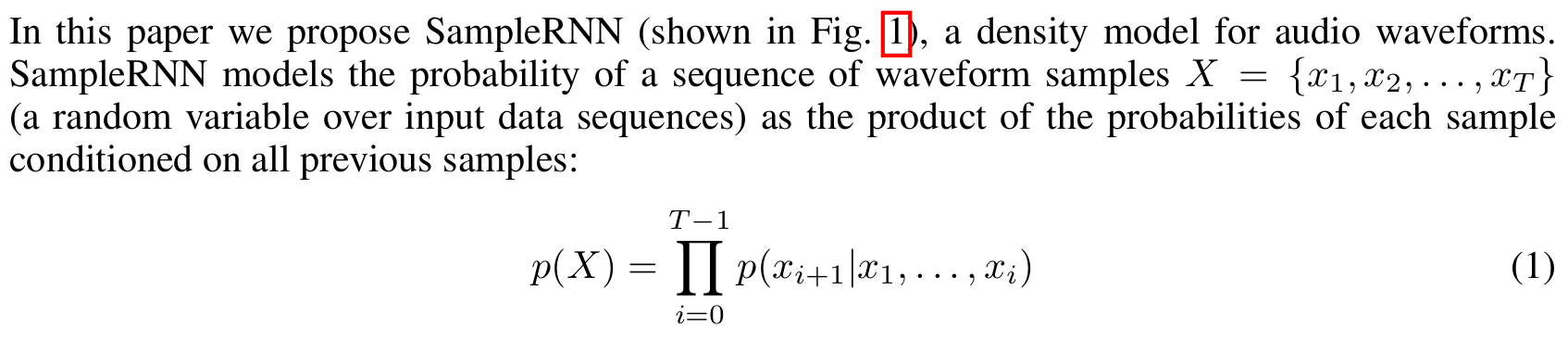A key concept in SampleRNN is the use of a hierarchy of modules operating at different temporal resolutions to capture the temporal patterns present in audio and music. This is related to how WaveNet used dilated convolutions to learn features at wider timescales than immediately consecutive samples.

The authors of SampleRNN also agree with WaveNet that discretizing the inputs gives better results than operating on the float samples directly:
We use a Softmax because we found that better results were obtained by discretizing the audio signals (also see van den Oord et al. (2016)) and outputting a Multinoulli distribution rather than using a Gaussian or Gaussian mixture to represent the conditional density of the original real-valued signal.
The reference implementation by the original authors of the paper, sampleRNN_ICLR2017, is less readable (to me) than the PRiSM-SampleRNN implementation, so I'll use the second for the code dissection.

## RNN primer

From the RNNoise website (expanded on more in the hybrid approach page of this project) comes a succint overview on the use of RNNs for modelling temporal sequences:

Recurrent neural networks (RNN) are very important here because they make it possible to model time sequences instead of just considering input and output frames independently. This is especially important for noise suppression because we need time to get a good estimate of the noise. For a long time, RNNs were heavily limited in their ability because they could not hold information for a long period of time and because the gradient descent process involved when back-propagating through time was very inefficient (the vanishing gradient problem). Both problems were solved by the invention of gated units, such as the Long Short-Term Memory (LSTM), the Gated Recurrent Unit (GRU), and their many variants.

RNNoise uses the Gated Recurrent Unit (GRU) because it performs slightly better than LSTM on this task and requires fewer resources (both CPU and memory for weights). Compared to simple recurrent units, GRUs have two extra gates. The reset gate controls whether the state (memory) is used in computing the new state, whereas the update gate controls how much the state will change based on the new input. This update gate (when off) makes it possible (and easy) for the GRU to remember information for a long period of time and is the reason GRUs (and LSTMs) perform much better than simple recurrent units.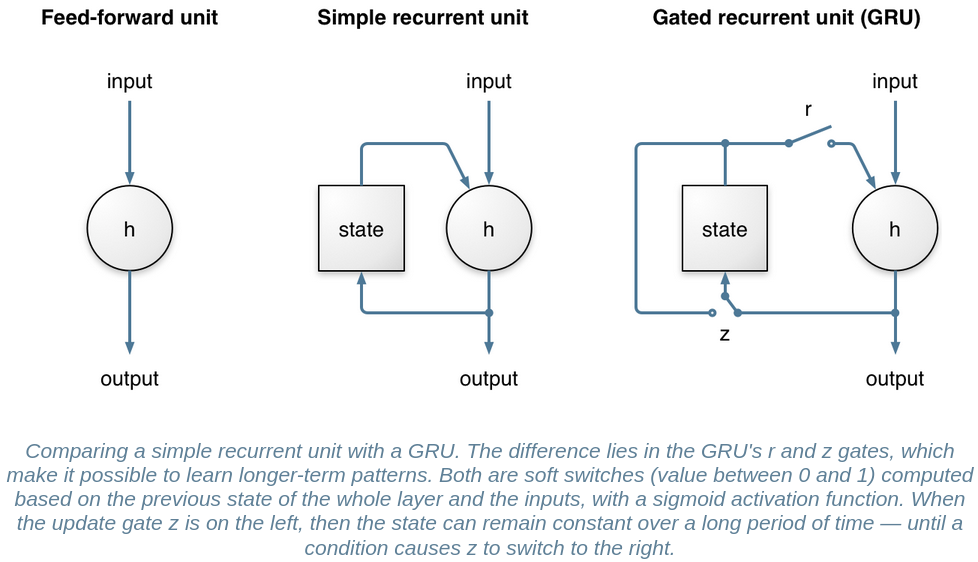## 2-tier vs. 3-tier SampleRNN

Something to note is that various sources mention that 2-tier SampleRNN creates better music than 3-tier SampleRNN:
There's also a 3-tier option, but we initially had better results with 2-tier, so we don't use 3-tier. It doesn't have the modifications we made to 2-tier.
Table 1 and Figure 4 also show the 2-tier SampleRNN outperforming the 3-tier model in terms of likelihood and human rating respectively, which is very counterintuitive as one would expect longer-range temporal correlations to be even more relevant for music than for speech. This is not discussed at all, I think it would be useful to comment on why this could be happening.
"Why 2-tier is outperforming the 3-tier model for music?" - We did not expect that, but for any dataset and architecture structure, there is an optimal depth. Considering that this is a deep RNN (which introduces a form of recurrent depth, here very large) and the hypothesis that it is difficult to train such architectures in the first place, it is possible that alternative training procedures could yield better results with a deeper model.

Recall that in the SampleRNN paper, the multiple tiers of the RNN determined the learning of audio patterns at different temporal scales. This is reflected in the following diagram from the paper: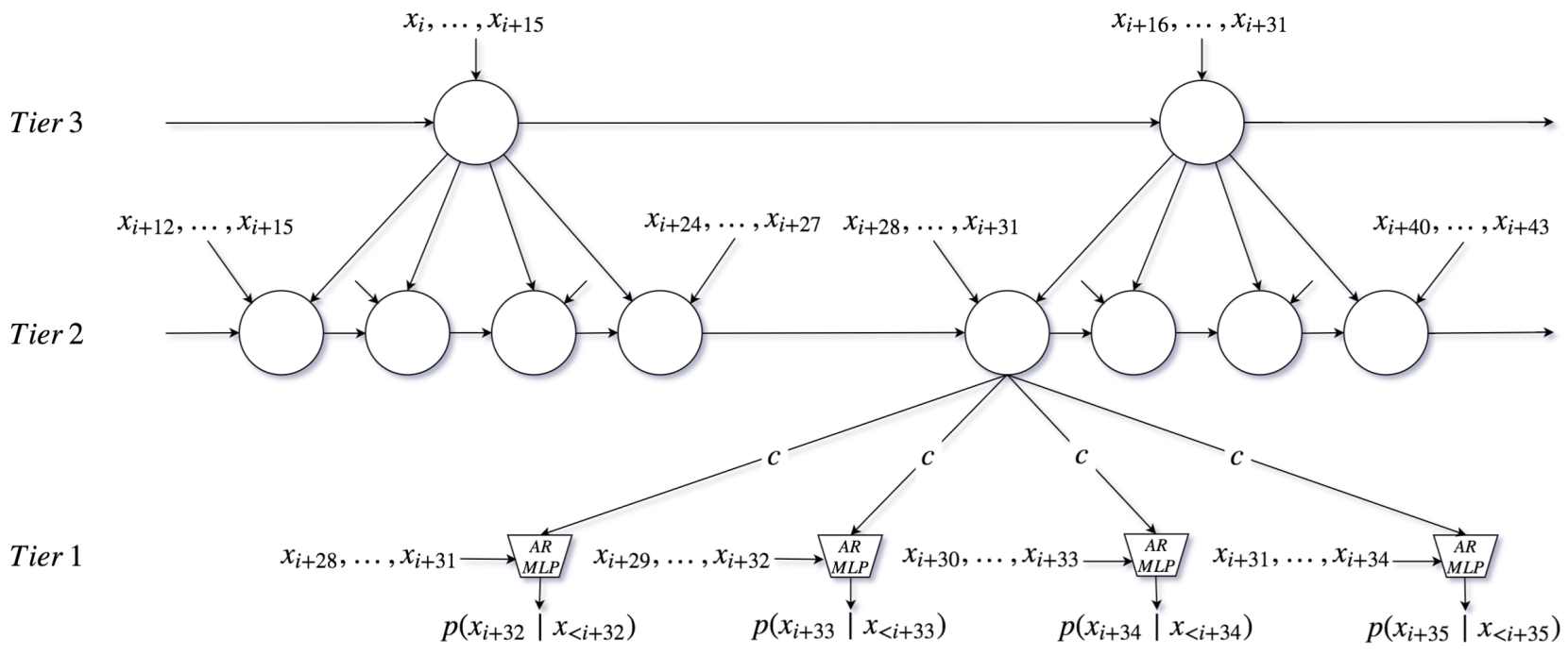Higher RNN tiers map to wider temporal scales 
The lowest temporal scale (consecutive samples) represents very low-level audio features (e.g. timbre), while higher scales can (hypothetically) go as far as representing repeating choruses or verses minutes apart. As such, it's interesting to note that 2-tiers, or only two temporal scales of learning, performed better than 3-tier, which should hypothetically be enforcing even longer-scale temporal patterns (and music has temporal patterns as coarse as minutes apart, e.g. a repeating chorus).

However, as the author says, 2-tier SampleRNN may have a depth that makes it more optimal considering the training architecture of SampleRNN (or in other words, there needs to be an analysis of alternative training architectures to make 3-tier beat 2-tier).

### Preprocessing

The preprocessing uses the same mu-law encoding as the WaveNet but without the one-hot encoding. The 256-bit quantized integer is used directly instead of converting it into a 256-length vector.

### Training layers - stack of hierarchical RNN modules

The SampleRNN model consists of 3 tiers:
• Sample MLP (in samplernn/sample_mlp.py)
• Frame RNN (in samplernn/frame_rnn.py)
• Big Frame RNN (in samplernn/frame_rnn.py)

#### Tier 1: Sample multilayer perceptron

The first tier is Sample MLP, which is a multilayer perceptron. The code for the Sample MLP is:
```    self.inputs = tf.keras.layers.Conv1D(
filters=self.dim, kernel_size=frame_size, use_bias=False
)
self.hidden = tf.keras.layers.Dense(self.dim, activation='relu')
self.outputs = tf.keras.layers.Dense(self.q_levels, activation='relu')
```
An MLP is a simpler, more primitive precursor to the more complex CNN (convolutional neural network like WaveNet) or RNN. This choice is described in the paper as saving computation cost since the sample-to-sample relationship among nearby samples is probably a simple one. They're implying that the more complex non-linearities of music are in the higher tiers, and that small local clusters of samples have a simpler relationships.

Interesting to note is that the Sample MLP takes conditioning (i.e. external influence) from the results of the Frame RNN one tier higher (in samplernn/sample_mlp.py - some parts omitted):
```    class SampleMLP(tf.keras.layers.Layer):

def __init__(self, frame_size, dim, q_levels, emb_size):
self.inputs = tf.keras.layers.Conv1D(
filters=self.dim, kernel_size=frame_size, use_bias=False
)
self.hidden = tf.keras.layers.Dense(self.dim, activation='relu')
self.outputs = tf.keras.layers.Dense(self.q_levels, activation='relu')

def call(self, inputs, conditioning_frames):
batch_size = tf.shape(inputs)

inputs = self.embedding(tf.reshape(inputs, [-1]))
inputs = self.inputs(tf.reshape(inputs, [batch_size, -1, self.q_levels]))

hidden = self.hidden(inputs + conditioning_frames)
return self.outputs(hidden)
```
Note how the externally-passed conditioning is added to the model's own hidden layers. Another notable feature is that the inputs to the MLP have a 1D convolution applied. This is not described in the paper, but a hybrid Conv1D-MLP model does exist in literature. It looks to me like in this implementation choice (PRiSM), they applied a hybrid Conv1D/MLP approach, especially since the reference SampleRNN implementation does not have any 1D convolutions in the SampleRNN code.

The summary of the first tier is that it establishes the lowest level of sample-to-sample relations in the input waveforms, just like the first 1D convolution of WaveNet.

I actually found support for MLP as first layer in a different paper:
As in the conventional 2D CNNs, the input layer is a passive layer that receives the raw 1D signal and the output layer is a MLP layer with the number of neurons equal to the number of classes
This seems to fit - the convention carried over from conventional 2D CNN models, since the SampleMLP has the same number of outputs as the quantization channels (256).

#### GRU and LSTM - different types of RNN

The SampleRNN authors used different choices (GRU and LSTM) of memory cell in the RNN, and concluded that the GRU performed best. Let's take a detour into this excellent overview paper on the use of GRU vs. LSTM gates in RNNs.

Recall that in the WaveNet overview, I talked about the vanishing gradient problem and how they used skip connections in the layer of convolutions to solve it. As the network gets deeper, small gradients tend to 0 and the model no longer knows how to improve. There need to be backward connections that jump several layers to connect the output closer to the input.

In a recurrent neural network, this backward memory to solve the vanishing gradient problem is solved by using LSTM (long short-term memory) units:
Unlike to the traditional recurrent unit which overwrites its content at each time-step (see Eq. (2)), an LSTM unit is able to decide whether to keep the existing memory via the introduced gates. Intuitively, if the LSTM unit detects an important feature from an input sequence at early stage, it easily carries this information (the existence of the feature) over a long distance, hence, capturing potential long-distance dependencies.
The introduction to GRU describes the importance of GRUs to capturing dependencies on different time scales:
A gated recurrent unit (GRU) was proposed by Cho et al.  to make each recurrent unit to adaptively capture dependencies of different time scale
The actual gated units resemble some gated logic processors: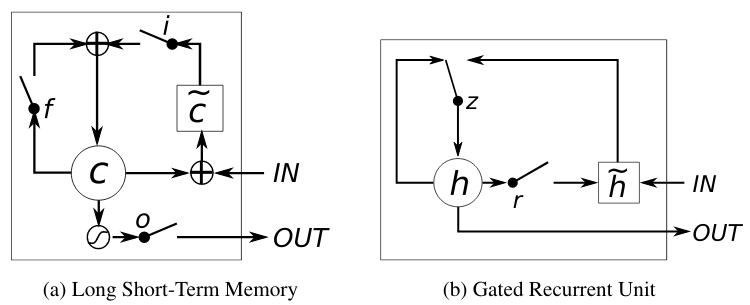In the WaveNet overview, I described how PixelCNN started using non-linear functions, sigmoid and tanh, to model non-linearities in the same way that an LSTM did in PixelRNN. Here, we come full circle and learn that the sigmoid and tanh functions are also implicated inside the implementation of LSTM and GRU units.

The authors conclude that both GRU- and LSTM-RNNs are an improvement over regular RNNs for modeling more complex relationships, and they demonstrate that GRUs train more efficiently.

#### Tier 2 and 3: Frame RNNs, frame size = 16,64

The next tiers are cascaded Frame RNNs with a frame size of 16 and 64 respectively (frame size 64 is referred to as the "big frame" in the code and paper). These are RNNs with the specified unit type (GRU in the default config, as mentioned):
```    class FrameRNN(tf.keras.layers.Layer):
def __init__(self, rnn_type, frame_size, num_lower_tier_frames, num_layers, dim, q_levels, skip_conn):
super(FrameRNN, self).__init__()
self.skip_conn = skip_conn
self.inputs = tf.keras.layers.Dense(self.dim)
self.rnn = RNN(rnn_type, self.dim, self.num_layers, self.skip_conn)

def build(self, input_shape):
self.upsample = tf.Variable(
tf.initializers.GlorotNormal()(
shape=[self.num_lower_tier_frames, self.dim, self.dim]),
name="upsample",
)

def call(self, inputs, conditioning_frames=None):
batch_size = tf.shape(inputs)

input_frames = tf.reshape(inputs, [
batch_size,
tf.shape(inputs) // self.frame_size,
self.frame_size
])
input_frames = ( (input_frames / (self.q_levels / 2.0)) - 1.0 ) * 2.0
num_steps = tf.shape(input_frames)

input_frames = self.inputs(input_frames)

if conditioning_frames is not None:
input_frames += conditioning_frames

frame_outputs = self.rnn(input_frames)
```
Interesting to note is that skip connections are used in the code but not mentioned in the paper. As covered, skip connections in WaveNet were to solve the vanishing gradient problem - but, in SampleRNN, the use of GRUs (or LSTMs) in the RNN should hypothetically solve the vanishing gradient problem. However, there is support in literature for skip RNNs - perhaps combining both brings even better performance.

Another thing to note is the upsampling of the samples to select samples that are spaced 16- and 64-samples apart for the small and big frames. This is exactly how WaveNet's dilated convolutions worked.

We also see that the FrameRNNs can be conditioned externally just like the first tier, Sample MLP. In practise, the big Frame RNN is unconditioned, but its decisions are used to condition the small Frame RNN and finally the Sample MLP. Putting it all together in samplernn/model.py:
```    class SampleRNN(tf.keras.Model):
def __init__(self, batch_size, frame_sizes, q_levels, q_type,
dim, rnn_type, num_rnn_layers, seq_len, emb_size, skip_conn):
super(SampleRNN, self).__init__()
self.big_frame_size = frame_sizes
self.frame_size = frame_sizes

self.big_frame_rnn = FrameRNN(
frame_size = self.big_frame_size,
)

self.frame_rnn = FrameRNN(
frame_size = self.frame_size,
)

self.sample_mlp = SampleMLP(
self.frame_size, self.dim, self.q_levels, self.emb_size
)
```

#### Generating audio

Following immediately from the last snippet, we can see the SampleRNN functions for creating a waveform:
```        # Inference
@tf.function
def inference_step(self, inputs, temperature):
num_samps = self.big_frame_size
samples = inputs
big_frame_outputs = self.big_frame_rnn(tf.cast(inputs, tf.float32))
for t in range(num_samps, num_samps * 2):
frame_inputs = samples[:, t - self.frame_size : t, :]
big_frame_output_idx = (t // self.frame_size) % (
self.big_frame_size // self.frame_size
)
frame_outputs = self.frame_rnn(
tf.cast(frame_inputs, tf.float32),
conditioning_frames=unsqueeze(big_frame_outputs[:, big_frame_output_idx, :], 1))
sample_inputs = samples[:, t - self.frame_size : t, :]
sample_outputs = self.sample_mlp(
sample_inputs,
conditioning_frames=unsqueeze(frame_outputs[:, frame_output_idx, :], 1))

def call(self, inputs, training=True, temperature=1.0):
if training==True:
# UPPER TIER
big_frame_outputs = self.big_frame_rnn(
tf.cast(inputs, tf.float32)[:, : -self.big_frame_size, :]
)
# MIDDLE TIER
frame_outputs = self.frame_rnn(
tf.cast(inputs, tf.float32)[:, self.big_frame_size-self.frame_size : -self.frame_size, :],
conditioning_frames=big_frame_outputs,
)
# LOWER TIER (SAMPLES)
sample_output = self.sample_mlp(
inputs[:, self.big_frame_size - self.frame_size : -1, :],
conditioning_frames=frame_outputs,
)
return sample_output
else:
return self.inference_step(inputs, temperature)
```
We can see the results of the 3 tiers cascading down into the lower tier, influencing the generation of the final waveform. The actual waveform results are created by the `call` functions of the Sample MLP and Frame RNNs shown above.

### Loss function

The loss function and training parameter optimization code is very similar to the WaveNet code, down to the optimizer factory. From `train.py`:
```    def create_adam_optimizer(learning_rate, momentum):
epsilon=1e-4)

def create_sgd_optimizer(learning_rate, momentum):
return tf.optimizers.SGD(learning_rate=learning_rate,
momentum=momentum)

def create_rmsprop_optimizer(learning_rate, momentum):
return tf.optimizers.RMSprop(learning_rate=learning_rate,
momentum=momentum,
epsilon=1e-5)

'sgd': create_sgd_optimizer,
'rmsprop': create_rmsprop_optimizer}

# Optimizer
opt = optimizer_factory[args.optimizer](
learning_rate=args.learning_rate,
momentum=args.momentum,
)

# Compile the model
compute_loss = tf.keras.losses.SparseCategoricalCrossentropy(from_logits=True)
train_accuracy = tf.keras.metrics.SparseCategoricalAccuracy(name='accuracy')
model.compile(optimizer=opt, loss=compute_loss, metrics=[train_accuracy])
```
These are passed into the SampleRNN model code (`samplernn/model.py`):
```    def train_step(self, data):
(x, y) = data
raw_output = self(x, training=True)
prediction = tf.reshape(raw_output, [-1, self.q_levels])
target = tf.reshape(y, [-1])
loss = self.compiled_loss(
target,
prediction,
regularization_losses=self.losses)
self.compiled_metrics.update_state(target, prediction)
return {metric.name: metric.result() for metric in self.metrics}
```
Like with WaveNet, a predicted waveform is produced from the model during training and then compared to the input waveforms to compute the loss. The actual prediction is done with `self(x, training=True)`, which in Python would be implemented by the object's `call()` function:
```    def call(self, inputs, training=True, temperature=1.0):
# UPPER TIER
big_frame_outputs = self.big_frame_rnn(
tf.cast(inputs, tf.float32)[:, : -self.big_frame_size, :]
)
# MIDDLE TIER
frame_outputs = self.frame_rnn(
tf.cast(inputs, tf.float32)[:, self.big_frame_size-self.frame_size : -self.frame_size, :],
conditioning_frames=big_frame_outputs,
)
# LOWER TIER (SAMPLES)
sample_output = self.sample_mlp(
inputs[:, self.big_frame_size - self.frame_size : -1, :],
conditioning_frames=frame_outputs,
)
return sample_output
```
The values are 16-sample frames for the middle tier, and 64-sample frames for the upper tier. Here we see a key distinction between SampleRNN and WaveNet. WaveNet uses the weights of the dilated convolution network to predict samples with knowledge of different temporal scales built in. SampleRNN is using patterns learned at broad temporal scales to condition the lower temporal scales - this means that SampleRNN's choice of high-level/long-term temporal feature feeds into the subsequent choices for the low-level temporal feature predictions.

The optimizers are the same. The loss function is in fact the same as WaveNet, except that WaveNet used TensorFlow 1's `softmax_cross_entropy_with_logits` function, while SampleRNN uses a slightly different API,`SparseCateoricalCrossentropy`. The difference is explained simply that if your data is one-hot encoded (i.e. 256-bit mu-law integers expanded into a vector of 256 0s or 1s, like WaveNet), you would use the softmax cross entropy function, whereas if they're integers (like SampleRNN), you would use a sparse softmax cross entropy function.

We can also see the input data is batched as in WaveNet, indicating similar use of mini-batch iterative training (aka mini-batch Stochastic Gradient Descent):
```    initial_epoch = get_initial_epoch(resume_from)
dataset = get_dataset(args.data_dir, args.num_epochs-initial_epoch, args.batch_size, seq_len, overlap)

# Dataset iterator
def train_iter():
for batch in dataset:
num_samps = len(batch)
for i in range(overlap, num_samps, seq_len):
x = quantize(batch[:, i-overlap : i+seq_len], q_type, q_levels)
y = x[:, overlap : overlap+seq_len]
yield (x, y)

callbacks = [
TrainingStepCallback(
model = model,
num_epochs = args.num_epochs,
steps_per_epoch = steps_per_epoch,
steps_per_batch = steps_per_batch,
ModelCheckpointCallback(
monitor = 'loss',
save_weights_only = True,
save_best_only = args.checkpoint_policy.lower()=='best',
save_freq = args.checkpoint_every * steps_per_epoch),
tf.keras.callbacks.EarlyStopping(
monitor = 'loss',
patience = args.early_stopping_patience),
]
```
The above code is the equivalent of the training loop of WaveNet, where the SampleRNN model exposes its trainable variables and the Tensorflow library is leveraged to use the loss function above to train the model.

Note a detail of SampleRNN is that they're using the keras EarlyStopping which stops training if it predicts that the model is not improving enough to be worthwhile. In WaveNet, the training proceeds for as many steps as the user requested.